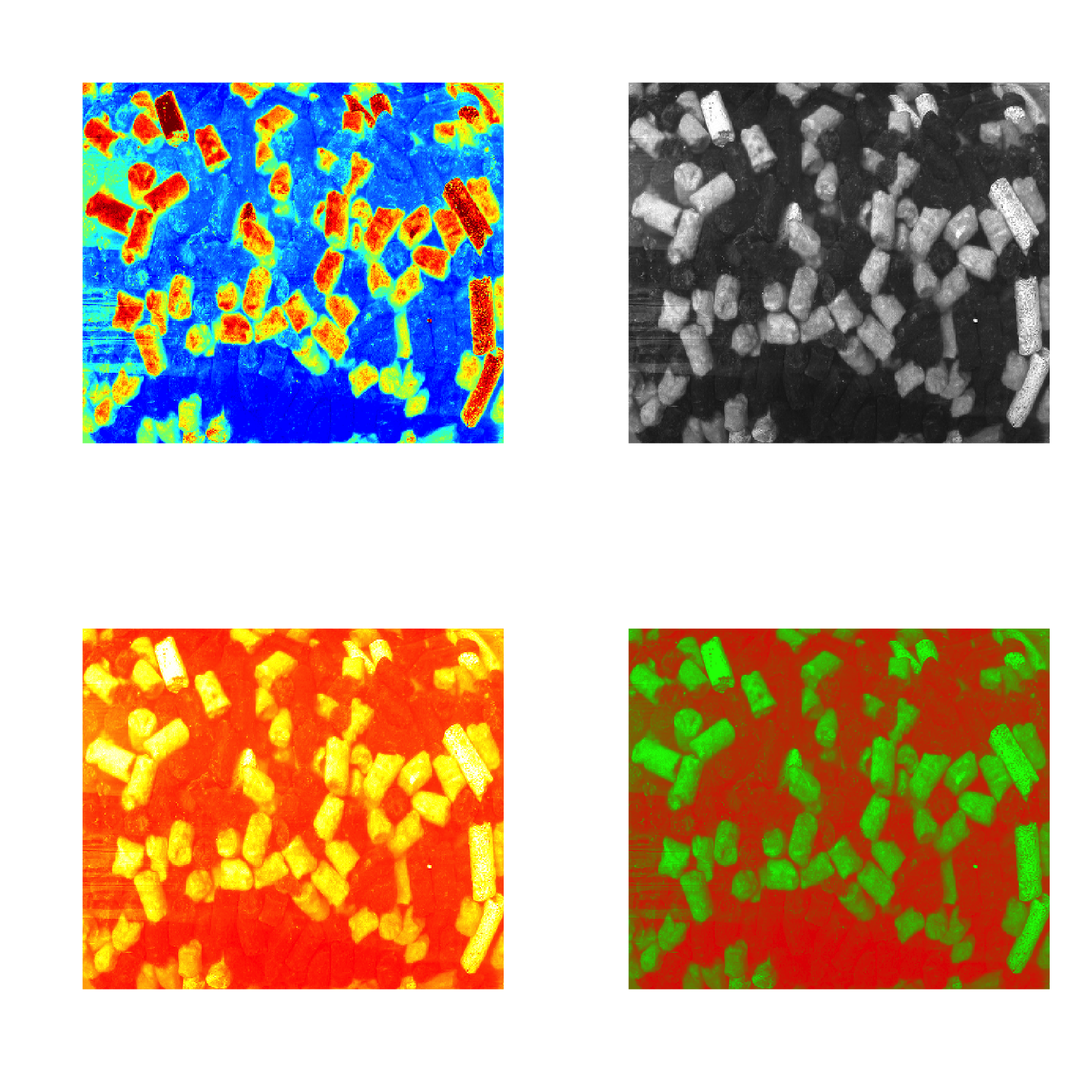## Autoscaling

Autoscaling consists of two steps. First step is centering (or, more precise, mean centering) when center of a data cloud in variable space is moved to an origin. Mathematically it is done by subtracting mean from the data values separately for every column/variable. Second step is scaling og standardization when data values are divided to standard deviation so the variables have unit variance. This autoscaling procedure (both steps) is known in statistics simply as standardization. You can also use arbitrary values to center or/and scale the data, in this case use sequence or vector with these values should be provided as an argument for center or scale.

R has a built-in function for centering and scaling, scale(). The method prep.autoscale() is actually a wrapper for this function, which is mostly needed to set all user defined attributes to the result (all preprocessing methods will keep the attributes). Here are some examples how to use it:

library(mdatools)

data(people)

# mean centering only
data1 = prep.autoscale(people, center = TRUE, scale = FALSE)

# scaling/standardization only
data2 = prep.autoscale(people, center = FALSE, scale = TRUE)

# autoscaling (mean centering and standardization)
data3 = prep.autoscale(people, center = TRUE, scale = TRUE)

# centering with median values and standardization
data4 = prep.autoscale(people, center = apply(people, 2, median), scale = TRUE)

par(mfrow = c(2, 2))
boxplot(data1, main = "Mean centered")
boxplot(data2, main = "Standardized")
boxplot(data3, main = "Mean centered and standardized")
boxplot(data4, main = "Median centered and standardized")The method has also an additional parameter max.cov which allows to avoid scaling of variables with zero or very low variation. The parameter defines a limit for coefficient of variation in percent sd(x) / m(x) * 100 and the method will not scale variables with coefficient of variation below this limit. Default value for the parameter is 0 which will prevent scaling of constant variables (which is leading to Inf values).